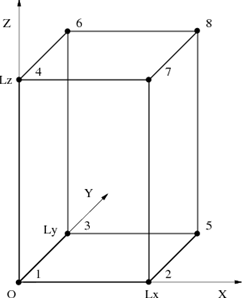Home > Resources > Making Animation with Scilab > 5. Creating 3D shape - Block (1)
Making Animation with Scilab

## 5. Creating 3D shape - Block (1)

Let's create a block shape by combining six polygons using `plot3d` command. We first consider the case where one of the vertices coincides with the origin of the coordinate system and each side is parallel to the coordinate axis as shown in Figure 1 (we call this the "basic configuration"). In this case, if the length of each side (Lx, Ly, Lz) is given, the coordinates of the eight vertices are obvious. The six faces are defined by the following six sequences of vertices.

• 1-2-5-3
• 1-3-6-4
• 1-4-7-2
• 4-7-8-6
• 2-5-8-7
• 3-6-8-5Figure 1: Block shape (in the basic configuration)

A Scilab script to draw a block shape in the basic configuration is shown in Code 1. The result of the drawing by this script is shown in Figure 2. Function `genpat()` used in the script is a function defined as in Code 2 that generates patches data, which is the input to `plot3d`, from vertices and faces matrices.

```// make_block_special.sce

clear; xdel(winsid());

exec('genpat.sci', -1);

// Side lengths
Lx = 0.15;
Ly = 0.05;
Lz = 0.30;

// Vertices
vertices = [
0,   0,  0;  // #1
Lx,  0,  0;  // #2
0,  Ly,  0;  // #3
0,   0, Lz;  // #4
Lx, Ly,  0;  // #5
0,  Ly, Lz;  // #6
Lx,  0, Lz;  // #7
Lx, Ly, Lz]; // #8

// Faces
faces = [
1, 2, 5, 3;  // #1
1, 3, 6, 4;  // #2
1, 4, 7, 2;  // #3
4, 7, 8, 6;  // #4
2, 5, 8, 7;  // #5
3, 6, 8, 5]; // #6

// Patches
patches = genpat(vertices, faces);

// Draw patches
h_fig = figure;
h_fig.background = 8;
h_pat = plot3d(patches.x, patches.y, patches.z);
h_pat.color_mode = 4;
h_pat.foreground = 1;
h_pat.hiddencolor = 4;

// Axes settings
xlabel("x"); ylabel("y"); zlabel("z");
h_axes = gca();
h_axes.isoview = "on";
h_axes.box = "off";
h_axes.rotation_angles = [63.5, -127];
h_axes.data_bounds = [-0.2, -0.2, -0.1; 0.4, 0.3, 0.4];
xgrid;
```
Code 1: Scilab script to draw a block shape (in the basic configuration)
```function patches = genpat(vertices, faces)
// Generate patches data from vertices and faces
patches.x = vertices(:,1)(faces');
patches.y = vertices(:,2)(faces');
patches.z = vertices(:,3)(faces');
endfunction
```
Code 2: Scilab function that generates patches data from vertices and faces of a shapeFigure 2: A block shape made of six polygons (in the basic configuration)